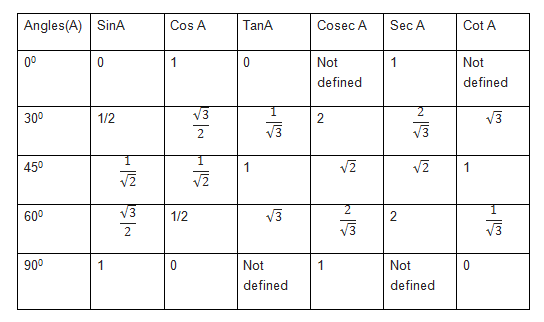# NCERT Solutions for Class 10 Maths Chapter 8: Introduction to Trigonometry Exercise 8.2

## NCERT Solutions for Class 10 Maths Introduction to Trigonometry Exercise 8.2

In this page we have NCERT Solutions for Class 10 Maths Chapter 8: Introduction to Trigonometry for EXERCISE 8.2 . Hope you like them and do not forget to like , social_share and comment at the end of the page.
Formula to RememberQuestion 1
Evaluate the following :
(i) $\sin 60^{\circ} \cos 30^{\circ} + \sin 30^{\circ} \cos 60^{\circ}$
(ii) $2 \tan^2 45^{\circ} + \cos^2 30^{\circ} - sin^2 60^{\circ}$
(iii)$\frac {\cos 45^{\circ}}{\sec 30^{\circ} + \csc 30^{\circ}}$
(iv) $\frac {\sin 30^{\circ} + \tan 45^{\circ} - \csc 60^{\circ}}{\sec 30^{\circ} + \cos 60^{\circ} + cot 45^{\circ}}$
(v) $\frac {5 \cos^2 60^{\circ}+ 4 \sec^2 30^{\circ}- \tan^2 45^{\circ}}{\sin^2 30^{\circ} + \cos^2 30^{\circ}}$
Solution
(i) $\sin 60^{\circ} \cos 30^{\circ} + \sin 30^{\circ} \cos 60^{\circ}$
$= ( \frac {\sqrt {3}}{2} \times \frac {\sqrt {3}}{2})+ ( \frac {1}{2} \times \frac {1}{2}) = \frac {3}{4}+ \frac {1}{4} = \frac {4}{4} = 1$
(ii) $2 \tan^2 45^{\circ} + \cos^2 30^{\circ} - sin^2 60^{\circ}$
$= 2 \times (1)^2 + (\frac {\sqrt {3}}{2})^2 - (\frac {\sqrt {3}}{2})^2= 2$

(iii) $\frac {\cos 45^{\circ}}{\sec 30^{\circ} + \csc 30^{\circ}}$

$= \frac {\frac {1}{\sqrt {2}}}{ \frac {2}{\sqrt {3}}+ 2}$
$= \frac {\sqrt {3}}{\sqrt {2} (2+2\sqrt {3})} = \frac {\sqrt {3}}{2 \sqrt {2} +2\sqrt {6}}$
$= \frac {\sqrt {3} (2\sqrt {6}-2 \sqrt {2})}{(2 \sqrt {2} +2\sqrt {6})(2\sqrt {6} -2 \sqrt {2})}$
$= \frac {\sqrt {3} (2\sqrt {6}-2 \sqrt {2})}{24-8} = \frac {2 \sqrt {3}(\sqrt {6}- \sqrt {2})}{16}$
$= \frac {\sqrt {3} (\sqrt {6}- \sqrt {2})}{8} = \frac {3 \sqrt {2}- \sqrt {6}}{8}$

(iv) $\frac {\sin 30^{\circ} + \tan 45^{\circ} - \csc 60^{\circ}}{\sec 30^{\circ} + \cos 60^{\circ} + cot 45^{\circ}}$
$= \frac {1/2 + 1- 2/\sqrt {3}}{2 /\sqrt {3} + 1/2 + 1}$
$= \frac {3/2 - 2/\sqrt {3}}{3/2 + 2/\sqrt {3}}$
$= \frac {3 \sqrt {3}-4}{3 \sqrt {3} +4}$
Similifying the fraction
$= \frac {(3 \sqrt {3}-4)(3 \sqrt {3}-4)}{(3 \sqrt {3} +4)(3 \sqrt {3}-4)}$
$= \frac {27+16 - 24 \sqrt {3}}{27-16}$
$= \frac {43-24 \sqrt {3}}{11}$

(v) $\frac {5 \cos^2 60^{\circ}+ 4 \sec^2 30^{\circ}- \tan^2 45^{\circ}}{\sin^2 30^{\circ} + \cos^2 30^{\circ}}$
$= \frac {5(1/2)^2+4(2/\sqrt {3})^2-1^2}{(1/2)^2+(\sqrt {3}/2)^2}$
$= \frac {5/4 + 16/3 -1}{1/4 + 3/4}$
$= \frac {15+64-12}{12}$
$= \frac {67}{12}$

Question 2
Choose the correct option and justify your choice :
(i) $\frac {2 \tan 30^{\circ}}{1+ \tan^2 30^{\circ}} =$
(A) sin 60°
(B) cos 60°
(C) tan 60°
(D) sin 30°

(ii) $\frac {1- \tan^2 45^{\circ}}{1+ \tan^2 45^{\circ}} =$
(A) tan 90°
(B) 1
(C) sin 45°
(D) 0

(iii) $\sin 2A = 2 \sin A$ is true when A =
(A) 0°
(B) 30°
(C) 45°
(D) 60°
(iv) $\frac {2 \tan30^{\circ}}{1- \tan^2 30^{\circ}} =$
(A) cos 60°
(B) sin 60°
(C) tan 60°
(D) sin 30°
Solution
(i)
$\frac {2 \tan 30^{\circ}}{1+ \tan^2 30^{\circ}} = \frac {2( \frac {1}{\sqrt {3}})}{1+(\frac {1}{\sqrt {3}})^2}$
$= \frac {6}{4 \sqrt {3}}= \frac {\sqrt {3}}{2} = \sin 60^{\circ}$
Hence (A) is correct.

(ii)
$\frac {1- \tan^2 45^{\circ}}{1+ \tan^2 45^{\circ}} = \frac {1-1^2}{1+1^2}=0$
Hence (D) is correct.

(iii)
$\sin 2A = 2 \sin A$
We know that only value satisfies this equation from option is 0 Hence (A) is correct.

(iv)
$\frac {2 \tan30^{\circ}}{1- \tan^2 30^{\circ}} = \frac {2(\frac {1}{\sqrt {3}})}{1-(\frac {1}{\sqrt {3}})^2}$
$= \sqrt {3}= \tan 60^{\circ}$
Hence (C) is correct.

Question 3
If $\tan (A + B) = \sqrt {3}$ and $\tan (A - B) = \frac {1}{\sqrt {3}}$ , $0^{\circ} < A + B \leq 90^{\circ}$ , A > B, find A and B.
Solution
$\tan (A + B) = \sqrt {3}$
Now we know that $\tan 60^{\circ} =\sqrt {3}$ Therefore
$\tan (A + B) = \tan 60^{\circ}$
or $(A + B) = 60^{\circ}$ --- (i)
Also
$\tan (A - B) = \frac {1}{\sqrt {3}}$
Now we know that $\tan 30^{\circ} =\frac {1}{\sqrt {3}}$ Therefore
$\tan (A - B) = \tan 30^{\circ}$
or $(A - B) = 30^{\circ}$ -- (ii)
Adding (i) and (ii), we get
$2A = 60^{\circ} + 30^{\circ}$
$A= 45^{\circ}$
Putting the value of A in equation (i)
$45^{\circ} + B = 60^{\circ}$
$B = 15^{\circ}$

Question 4
(i) $\sin (A + B) = \sin A + \sin B$.
(ii) The value of $\sin \theta$ increases as $\theta$ increases.
(iii) The value of $\cos \theta$ increases as $\theta$ increases.
(iv) $\sin \theta = \cos \theta$ for all values of $\theta$.
(v) $\cot A$ is not defined for A = 0°.
Solution
(i) False.
Let A = 30° and B = 60°, then
$\sin (A + B) = \sin (30^{\circ} + 60^{\circ})= \sin 90^{\circ}= 1$ and,
$\sin A + \sin B = \sin 30^{\circ} + \sin 60^{\circ}$
$= \frac {1}{2} + \frac {\sqrt {3}}{2} = \frac {1+ \sqrt {3}}{2}$

(ii) True.
sin 0° = 0 < sin 30° = 1/2 < sin 45° = 1/√2 < sin60° =√3/2 < sin 90° = 1
Thus the value of sin θ increases as θ increases.

(iii) False.
cos 0° = 1 > cos 30° = √3/2 > cos 45° = 1/√2 > cos 60° =1/2 > cos 90° = 0
Thus the value of cos θ decreases as θ increases.

(iv) True.
We know that
$\cot A = \frac {\cos A}{\sin A}$
Therefore
$\cot 0^{\circ}= \frac {\cos 0^{\circ}}{\sin 0^{\circ}} =\frac { 1}{0} = \text {undefined}$.Go back to Class 10 Main Page using below links

### Practice Question

Question 1 What is $1 - \sqrt {3}$ ?
A) Non terminating repeating
B) Non terminating non repeating
C) Terminating
D) None of the above
Question 2 The volume of the largest right circular cone that can be cut out from a cube of edge 4.2 cm is?
A) 19.4 cm3
B) 12 cm3
C) 78.6 cm3
D) 58.2 cm3
Question 3 The sum of the first three terms of an AP is 33. If the product of the first and the third term exceeds the second term by 29, the AP is ?
A) 2 ,21,11
B) 1,10,19
C) -1 ,8,17
D) 2 ,11,20